Home
Previous — 36° system, part two
A thirty-degree system.
Version of 28 October 2010.

28. Introduction. In the 60° and 90° systems, it is easy to find the right length of track to fill a gap. Because the greatest common factor of 60 and 90 is 30, one might ask whether a 30° scheme would itself be simple to use. This page addresses the question.

Although overall track width will still be 40mm, circular segments will now be of radius 300mm rather than 200mm. This change is necessary because for a common configuration of switch, a curved segment of radius 200mm will not clear the straight segment. Figure 28-1 illustrates the problem and a solution.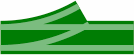Figure 28-1a200mm radius;unacceptable Figure 28-1b300mm radius;acceptable scale 4x

The minimum radius to make this switch work is 278.56mm [=140 + 80 × √3], and 300 is a convenient round number. With the 300mm radius, the minimum length of the straight segment is 154.92mm [= √(3202 − 2802)], or the straight segment will not clear the curved segment.

In the 30° system, there are twelve segments to a circle. Figure 28-2 contrasts the 200 and 300mm radii.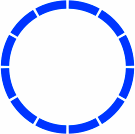Figure 28-2a200mm radius Figure 28-2b300mm radius

Corresponding to figure 16-2 is 28-3, which shows that a 30° system will need a straight segment 600mm long, or some equivalent combination of shorter segments.

Figure 28-3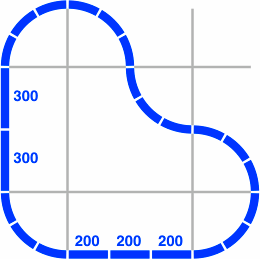Figure 28-4 contains in gray a 30°-60°-90° triangle, similar in role to figures 20-5 and 20-6 of the 36° system.

Figure 28-4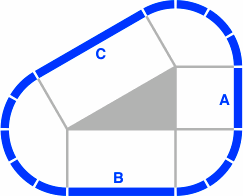Elementary trigonometry applied to figure 28-4 says that the length of side C must be twice the length of side A, while the ratio of B to A is √3, approximately 1.73205. Each column of table 28-A gives a few examples of what lengths the three sides can assume. Each number, representing millimeters, is rounded to the nearest integer.

Table 28-A
Sides of some triangles
A50657587100130150173200260300346
B87112130150173225260300346450520600
C100130150173200260300346400520600693

Why can there be so many track lengths in the 30° system? To answer this, we can start with 600mm, which according to figure 28-3 needs to be an attainable length:

• If A = 600, then B = 1039 and C = 1200
• If B = 600, then C = 693 and A = 346
• If C = 600, then A = 300 and B = 520
But new segment lengths can be generated by any of these actions:
• placing 1039 or 520 in position A or C
• placing 1200 or 693 in position A or B
• placing 300 or 346 in position B or C

Those lengths will in turn propagate a third round of lengths, et cetera.

The relation of C and A means that given any length, it will need to either doubled or halved to finish the triangle. Similarly, the relation of B and A means that any length requires multiplying or dividing by √3. As a result, a comprehensive table of segment lengths is two-dimensional.

Table 28-B gives the big picture of how the numbers of table 28-A are calculated. Within each box, above the line, are an integer approximation (in bold), a two-place approximation, and an exact expression. Below the line are found two-way and three-way decompositions. The arbitrary starting point is 600 (in the shaded box), from figure 28-2.

Table 28-B
Some track lengths
2−3 2−2 2−1 20 2+1
3−1.0 25
25.00
600 × 2−3 × 3−1.0
13 + 13
8 + 8 + 8
50
50.00
600 × 2−2 × 3−1.0
25 + 25
17 + 17 + 17
100
100.00
600 × 2−1 × 3−1.0
50 + 50
33 + 33 + 33
200
200.00
600 × 20 × 3−1.0
100 + 100
67 + 67 + 67
400
400.00
600 × 2+1 × 3−1.0
200 + 200
133 + 133 + 133
3−0.5 43
43.30
600 × 2−3 × 3−0.5
22 + 22
14 + 14 + 14
87
86.60
600 × 2−2 × 3−0.5
43 + 43
29 + 29 + 29
173
173.21
600 × 2−1 × 3−0.5
87 + 87
58 + 58 + 58
346
346.41
600 × 20 × 3−0.5
173 + 173
115 + 115 + 115
693
692.82
600 × 2+1 × 3−0.5
346 + 346
231 + 231 + 231
30 75
75.00
600 × 2−3 × 30
38 + 38
25 + 25 + 25
150
150.00
600 × 2−2 × 30
75 + 75
50 + 50 + 50
300
300.00
600 × 2−1 × 30
150 + 150
100 + 100 + 100
600
600.00
600 × 20 × 30
300 + 300
200 + 200 + 200
1200
1200.00
600 × 2+1 × 30
600 + 600
400 + 400 + 400
3+0.5 130
129.90
600 × 2−3 × 3+0.5
65 + 65
43 + 43 + 43
260
259.81
600 × 2−2 × 3+0.5
130 + 130
87 + 87 + 87
520
519.62
600 × 2−1 × 3+0.5
260 + 260
173 + 173 + 173
1039
1039.23
600 × 20 × 3+0.5
520 + 520
346 + 346 + 346
2078
2078.46
600 × 2+1 × 3+0.5
1039 + 1039
693 + 693 + 693

The table is two-dimensional in structure, in contrast to the primary series of the 45° system and the alpha series of the 36° system, which are one-dimensional.

Feasible lengths run from about 40mm to 800mm, but others are included to make the pattern clearer; the table can easily be extended to higher or lower powers of 2 or √3. Such extension will however reveal the inconvenient fact that, because √3 ÷ 2 is irrational, the quantity of lengths that can be produced in the feasible range is unbounded. Even more, the set of numbers obtainable by extending table 28-B are dense among the reals. What this means is that any nonnegative real number can be approximated arbitrarily closely by a number of the form 600 × 2m × 3n÷2, with m and n integer.

Of some mitigation is that any segment may be partitioned into two or three equal lengths: this aids in reducing the number of different lengths needed for practical use. Unfortunately, no small selection of the possible values is conspicuous as a working subset.

29. Figure-eights. Of these, there are five that are fundamental.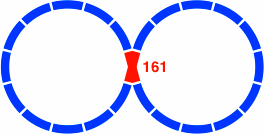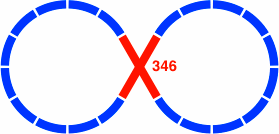Figure 29-111 curved segments per lobe 161 = 600 × tan15° ≠ 600 × 2m × 3n÷2 Figure 29-210 curved segments per lobe 346 = 600 × tan30° = 600 × 20 × 3−0.5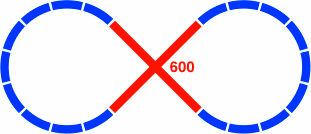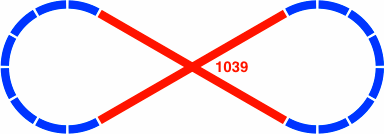Figure 29-39 curved segments per lobe 600 = 600 × tan45° = 600 × 20 × 30 Figure 29-48 curved segments per lobe 1039 = 600 × tan60° = 600 × 20 × 3+0.5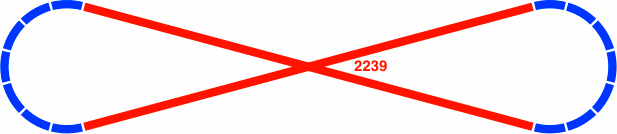Figure 29-57 curved segments per lobe 2239 = 600 × tan75° = 600 × 2+1 × 30 + 600 × 20 × 3+0.5

The following layouts substitute shorter segments for longer ones: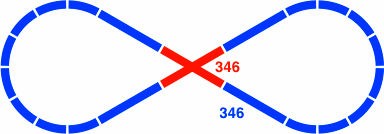Figure 29-6variant of 29-3 Figure 29-7variant of 29-4

The crossing piece in 29-7 is the same as that of 29-2. Although not illustrated, similar decompositions can be devised for the layout of figure 29-5 because 2239 = 1200 + 1039, both of which addends are found in table 28-B. An example is the breakdown 2239 = 1039 + 161 + 1039, which uses the same crossing as 29-1.

The length of the segments in the crossing of figure 29-1 is not an entry in table 28-B; rather, it is the difference between two entries: 161 = 1200 − 1039. Whether this distance might be the sum of finitely many entries is not yet known. In any case, pieces much shorter would not clear each other, because the minimum length is 149.28mm [= 40 ÷ tan15°].

Home
Previous — 36° system, part two